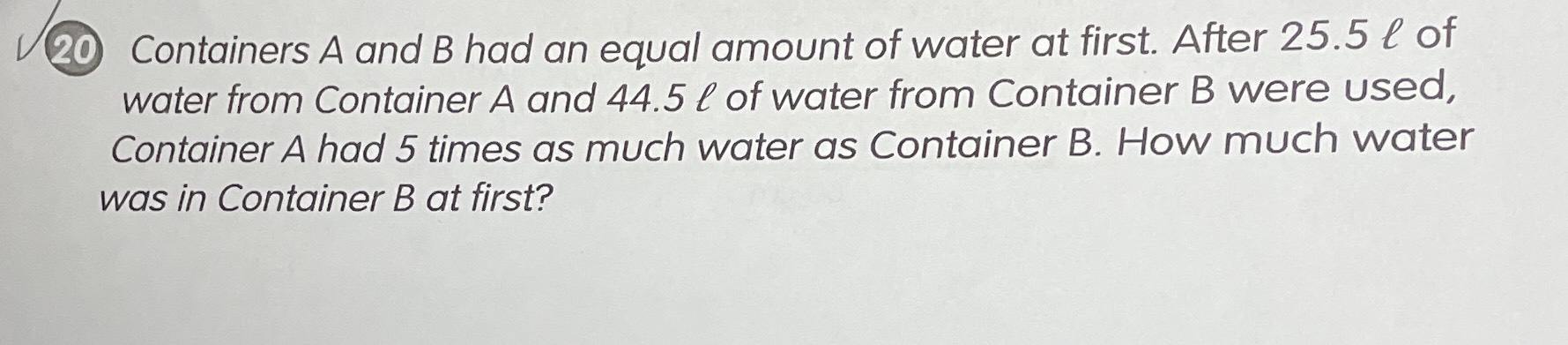### ¿Todavía tienes preguntas de matemáticas?

Pregunte a nuestros tutores expertos
Algebra
Pregunta20 Containers $$A$$ and $$B$$ had an equal amount of water at first. After $$25.5 l$$ of water from Container $$A$$ and $$44.5 \ell$$ of water from Container B were used, container $$A$$ had $$5$$ times as much water as Container B. How much water was in Container $$B$$ at first?

$$x - 25.5 = 5 ( x - 44.5 ) : x = \frac { 197 } { 4 } ($$ Decimal: $$x = 49.25 )$$

$$x - 25.5 = 5 ( x - 44.5 )$$

Expand $$5 ( x - 44.5 ) : 5 x - 222.5$$

$$x - 25.5 = 5 x - 222.5$$ Add $$25.5$$ to both sides

$$x - 25.5 + 25.5 = 5 x - 222.5 + 25.5$$

Simplify

$$x = 5 x - 197$$

Subtract $$5 x$$ from both sides

$$x - 5 x = 5 x - 197 - 5 x$$

Simplify

$$- 4 x = - 197$$ Divide both sides by $$- 4$$

$$\frac { - 4 x } { - 4 } = \frac { - 197 } { - 4 }$$

Simplify

$$x = \frac { 197 } { 4 }$$

At first container B has 49.25 liters of water

Steps

Solución
View full explanation on CameraMath App.